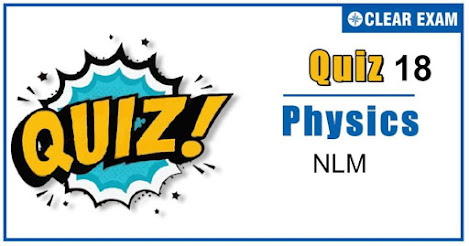## [LATEST]\$type=sticky\$show=home\$rm=0\$va=0\$count=4\$va=0

As per analysis for previous years, it has been observed that students preparing for NEET find Physics out of all the sections to be complex to handle and the majority of them are not able to comprehend the reason behind it. This problem arises especially because these aspirants appearing for the examination are more inclined to have a keen interest in Biology due to their medical background. Furthermore, sections such as Physics are dominantly based on theories, laws, numerical in comparison to a section of Biology which is more of fact-based, life sciences, and includes substantial explanations. By using the table given below, you easily and directly access to the topics and respective links of MCQs. Moreover, to make learning smooth and efficient, all the questions come with their supportive solutions to make utilization of time even more productive. Students will be covered for all their studies as the topics are available from basics to even the most advanced. .

Q1. Two blocks of equal masses m are released from the top of a smooth fixed wedge as shown in the figure
The acceleration of the centre of mass of the two blocks is :
•  g
•  g/2
•  3g/4
•  g/√2
Solution
The acceleration of the centre of mass of the block, =g/2 √((sin⁡∠ABC)2+(sin⁡∠ABC)2 ) =g/2 √(sin2 30°+sin2 60°) =g/2 √((0.5)2+(0.866)2 )=g/2

Q2. A given object taken n times as much time to slide down a 45° rough incline as it takes to slide down a perfectly smooth 45° incline. The coefficient of kinetic friction between the object and the incline is given by
•  (1-1/n2 )
•  1/(1-n2 )
•  √((1-1/n2 ) )
•  √(1/(1-n2 ))
Solution
μ= tan⁡θ (1-1/n2 )=1-1/n2 [As θ=45°]

Q3. The minimum force required to move a body up an inclined plane is three times the minimum force required to prevent it from sliding down the plane. If the coefficient of friction between the body and the inclined plane is 1/(2√3), the angle of the inclined plane is
•  60°
•  45°
•  30°
•  15°
Solution
Minimum force required to move a body up a rough inclined plane F1=mg(sin⁡θ+μ cos⁡θ) Minimum force required to prevent the body from sliding down the rough inclined plane. F2=μ mg cos⁡θ According to question F1=3 F2 ∴ mg(sin⁡θ+μ cos⁡θ)=3(μ mg cos⁡θ) sin⁡θ+μ cos⁡θ=3μ cos⁡θ sin⁡θ=2μ cos⁡θ tan⁡θ=2μ=2×1/(2√3)=1/√3=tan⁡30° θ=30°

Q4. Two blocks of massesm and 2m are connected by a light string passing over a frictionless pulley. As shown in the figure, the mass m is placed on a smooth inclined plane of inclination 30° and 2m hangs vertically. If the system is released, the blocks move with an acceleration equal to
•  g/4
•  g/3
•  g/2
•  g
Q5. At a certain instant of time the mass of rocket going up vertically is 100 kg. If it is ejecting 5 kg of gas per second at a speed of 400 m/s, the acceleration of the rocket would be (Taking g=10 m/s2).
•  20 m/s2
•  10 m/s2
•  2 m/s2
•  1 m/s2
Solution
The acceleration of a rocket is given by a=v/m (∆m/∆t)-g =400/100 [5/1]-10 =20-10=10 m/s2

Q6. A body presses a book against the front wall such that the book does not move. The force of friction between the wall and the book is?
•  Towards right
•  Towards left
•  Downwards
•  Upwards
Solution
The various forces acting on the book are as shown in the figure
Where, f= Frictional force W= Weight of a book F= Applied force N= Normal reaction From figure, The direction of force of friction between the wall and the book is upwards .

Q7. Impulse is
•  A scalar
•  Equal to change in the momentum of a body
•  Equal to rate of change of momentum of a body
•  A force
Solution
Impulse is given by the product of force and time. Form Newton’s second law F=ma=m ∆v/∆t F∆t=m∆v = change in the momentum of the body

Q8. A particle moves in a circular path with decreasing speed. Choose the correct statement
•  Angular momentum remains constant
•  Acceleration a->is towards the centre
•  Particle moves in a spiral path with decreasing radius
•  The direction of angular momentum remains constant
Solution
Angular momentum is an axial vector, so its direction is along the axis, perpendicular to the plane of motion which is not changing because of change of speed. Therefore, the direction of angular momentum remains the same and its magnitude may vary

Q9. Observer O1 is in a lift going upwards and O2 is on the ground. Both apply Newton’s law, and measure normal reaction on the body
•  Both measure the same value
•  Both measure zero
•  Both measure different value
•  No sufficient data
Solution
The value measured by O1 in N1=mg because acceleration of body with respect to O1 is zero The value measured by O2 is N2-mg=ma0 ∴ N2=m(g+a0) So, N1≠N2

Q10. A wooden box of mass 8 kg slides down an inclined plane of inclination 30° to the horizontal with a constant acceleration of 0.4 ms-2. What is the force of friction between the box and inclined plane? [g=10 ms-2]:
•  36.8 N
•  76.8 N
•  65.6 N
•  97.8 N
Solution
ma=mg sin⁡θ-f or f=mg sin⁡θ-ma =8[10×1/2-0.4] N = 8×4.6N = 36.8 N## Want to know more

Please fill in the details below:

## Latest NEET Articles\$type=three\$c=3\$author=hide\$comment=hide\$rm=hide\$date=hide\$snippet=hide

Name

ltr
item
BEST NEET COACHING CENTER | BEST IIT JEE COACHING INSTITUTE | BEST NEET, IIT JEE COACHING INSTITUTE: NLM Quiz 18
NLM Quiz 18
https://1.bp.blogspot.com/-SDEhNPi9tZ0/X5WwohTElTI/AAAAAAAABkU/L0ssaFmd3HIdZigG4Slexl3SI_za4ogRwCLcBGAsYHQ/w469-h246/Quiz%2BImage%2BTemplate%2B%252817%2529.jpg
https://1.bp.blogspot.com/-SDEhNPi9tZ0/X5WwohTElTI/AAAAAAAABkU/L0ssaFmd3HIdZigG4Slexl3SI_za4ogRwCLcBGAsYHQ/s72-w469-c-h246/Quiz%2BImage%2BTemplate%2B%252817%2529.jpg
BEST NEET COACHING CENTER | BEST IIT JEE COACHING INSTITUTE | BEST NEET, IIT JEE COACHING INSTITUTE
https://www.cleariitmedical.com/2020/10/NLMQuiz18.html
https://www.cleariitmedical.com/
https://www.cleariitmedical.com/
https://www.cleariitmedical.com/2020/10/NLMQuiz18.html
true
7783647550433378923
UTF-8